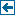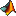Master index Index for chronux/locfit/Book# Index for chronux/locfit/Book

## Matlab files in this directory:fig10_1 Local Regression and Likelihood, Figure 10.1.fig11_1 Local Regression and Likelihood, Figure 11.1fig11_4 Local Regression and Likelihood, Figure 11.4.fig11_5 Local Regression and Likelihood, Figure 11.5.fig13_1 Local Regression and Likelihood, Figure 13.1fig1_1 Local Regression and Likelihood, Figure 1.1.fig1_2 Local Regression and Likelihood, Figure 1.2.fig2_2 Local Regression and Likelihood, Figure 2.2.fig2_3 Local Regression and Likelihood, Figure 2.3.fig2_4 Local Regression and Likelihood, Figure 2.4.fig2_5 Local Regression and Likelihood, Figure 2.5.fig2_6 Local Regression and Likelihood, Figure 2.6.fig2_7 Local Regression and Likelihood, Figure 2.7.fig3_1 Local Regression and Likelihood, Figure 3.1.fig4_1 Local Regression and Likelihood, Figure 4.1.fig4_2 Local Regression and Likelihood, Figure 4.2.fig4_3 Local Regression and Likelihood, Figure 4.3.fig4_4 Local Regression and Likelihood, Figure 4.4.fig5_1 Local Regression and Likelihood, Figure 5.1.fig5_2 Local Regression and Likelihood, Figure 5.2.fig5_3 Local Regression and Likelihood, Figure 5.3.fig5_4 Local Regression and Likelihood, Figure 5.4.fig5_5 Local Regression and Likelihood, Figure 5.5.fig5_6 Local Regression and Likelihood, Figure 5.6.fig6_1 Local Regression and Likelihood, Figure 6.1.fig6_2 Local Regression and Likelihood, Figure 6.2.fig6_3 Local Regression and Likelihood, Figure 6.3.fig6_4 Local Regression and Likelihood, Figure 6.4.fig6_5 Local Regression and Likelihood, Figure 6.5.fig6_6 Local Regression and Likelihood, Figure 6.6.fig6_7 Local Regression and Likelihood, Figure 6.6.fig7_1 Local Regression and Likelihood, Figure 7.1.fig7_2 Local Regression and Likelihood, Figure 7.2.fig7_3 Local Regression and Likelihood, Figure 7.3.fig7_4 Local Regression and Likelihood, Figure 7.4.fig7_5 Local Regression and Likelihood, Figure 7.5.fig7_6 Local Regression and Likelihood, Figure 7.6.fig8_1 Local Regression and Likelihood, Figure 8.1.fig8_2 Local Regression and Likelihood, Figure 8.2.fig8_3 Local Regression and Likelihood, Figure 8.3.fig9_1 Local Regression and Likelihood, Figure 9.1.fig9_2 Local Regression and Likelihood, Figure 9.2.runbook

## Other Matlab-specific files in this directory:

• border.mat
• cltest.mat
• cltrain.mat
• co2.mat
• ethanol.mat
• geyser.mat
• heart.mat
• iris.mat
• livmet.mat
• mcyc.mat
• mine.mat
• mmsamp.mat
• morths.mat
• penny.mat
• spencer.mat
• stamp.mat
• trimod.mat

Generated on Fri 28-Sep-2012 12:34:27 by m2html © 2005